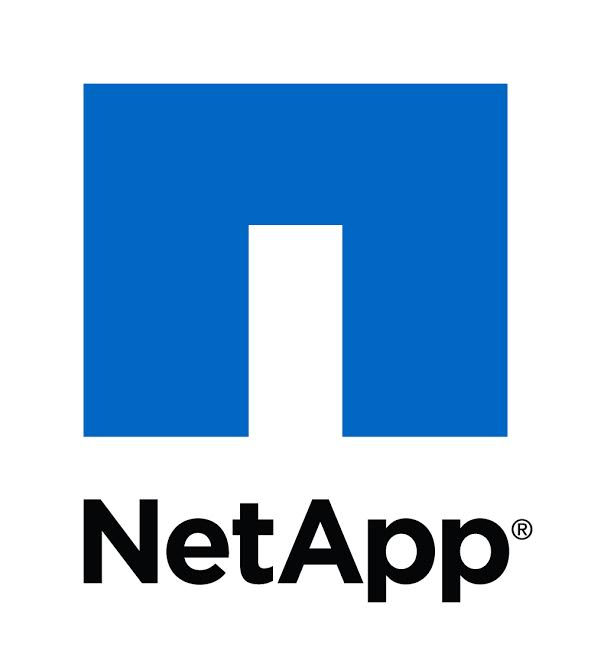Number Sum
Tag(s):

## Algorithms, Brute-force search, Dynamic Programming, Easy, Implementation, Introduction to Dynamic Programming 1

Problem
Editorial
Analytics

Alice is given an array of $N$ integers. Now, she is playing a game with Bob. In one turn Alice picks a sub-array of the given array $A$. In every turn, she picks a new subarray i.e. one with either different starting index or different ending index from the subarrays picked before.

Now, Bob starts shouting the natural numbers from beginning i.e. he first says $1$ then $2$ and so on. Alice stops him at the first integer which does not appear in the subarray that she has picked in this turn. Later on, she asks Bob to write the sum of all the values in which she stops him on a paper. Bob is weak in maths, can you help him?

Note: Please go through the sample carefully.

Input Format

The first line contains a single integer $N$. The next line contains $N$ space-separated integers, where the $i$-th integer denotes $A[i]$.

Output Format

Print the single integer representing the required result.

Constraints

$1 \le N \le 5 000$
$1 \le A[i] \le 10^9$

SAMPLE INPUT
3
1 2 3
SAMPLE OUTPUT
12
Explanation

Since, Alice can pick any subarray in any order. So let's find out the answer for each subarray and then add it.

Array = $[1\;2\;3]$

In the below lines $[a , b]$ denotes the subarray that inclues all elements in the range $[a , b]$:

$[1 , 1] = 2$
$[1 , 2] = 3$
$[1 , 3] = 4$
$[2 , 2] = 1$
$[2 , 3] = 1$
$[3 , 3] = 1$

So, the answer is $2 + 3 + 4 + 1 + 1 + 1 = 12$

Time Limit: 1.0 sec(s) for each input file.
Memory Limit: 256 MB
Source Limit: 1024 KB
Marking Scheme: Marks are awarded when all the testcases pass.
Allowed Languages: Bash, C, C++, C++14, Clojure, C#, D, Erlang, F#, Go, Groovy, Haskell, Java, Java 8, JavaScript(Rhino), JavaScript(Node.js), Julia, Kotlin, Lisp, Lisp (SBCL), Lua, Objective-C, OCaml, Octave, Pascal, Perl, PHP, Python, Python 3, R(RScript), Racket, Ruby, Rust, Scala, Swift, Swift-4.1, TypeScript, Visual Basic

## CODE EDITOR

Initializing Code Editor...
Your Rating:

## This Problem was Asked inChallenge Name

CodeNet 2018

OTHER PROBLEMS OF THIS CHALLENGE
• Algorithms > Dynamic Programming
• Algorithms > Graphs
• Data Structures > Advanced Data Structures
Notifications

?